### Electric Current Class 10th Physics & Chemistry AP Board Solution

##### Question 1.Explain how electron flow causes electric current with Lorentz- Drude theory of electrons.Answer:According to Drude and Lorentz conductors like metals contain a large number of free electrons while the positive ions are fixed in their position. In an open circuit in a conductor electrons move randomly so net charge is zero. But in closed circuit the electrons have an ordered motion, so there is a net charge crossing through any cross section of the conductor thus causes an electric current to flow.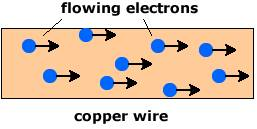Question 2.How does a battery work? Explain.Answer:i. When a conducting wire is connected to the terminals of the battery, a potential difference is created between the ends of the conductor.ii. This potential difference sets up an electrical field throughout the conductor. The conductor contains large number of electrons.iii. The electrons near the positive terminal of battery are attracted by it and start to move towards positive terminal.iv. As a result, the amount of positive charge on the plate decreases.v. So, the electric force becomes weaker than chemical force and the chemical force pulls negative ions from the positive plate (anode) and makes them move towards the negative plate (cathode).vi. The negative terminal pushes one electron into the conductor because of stronger repulsion between the negative terminal and negative ion.vii. Hence, the total number of electrons in the conductor remains constant during the current flow.viii. The above process continues till equilibrium is attained between electric force and chemical force.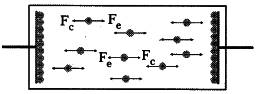Question 3.White the difference between potential difference and emf.Answer:Potential difference is the work done by the electric force on unit positive charge to move it through a distance l from point A to B is called potential difference between these two points. V = W/q = fel/qWhere V = potential difference;W = work done by the electric forceq = free chargel = distancefe = force exerted by the electric fieldThe work done by the chemical force to move unit positive charge from negative terminal to positive terminal of the battery is called electromotive force.EMF = W/q = Fd/qQuestion 4.How can you verify that the resistance of a conductor is temperature dependent?Answer:a. A bulb was taken and its resistance was measured using a multimeter in open circuit.b. The bulb is connected to a circuit and switch is put on.c. The bulb glows and after few minutes it gets heated up.d. Again the resistance of the bulb is measured using a multimeter.e. Value of resistance is found to be more in the second case than in the first case.f. As the temperature of the filament in bulb increases the resistance of bulb also increases.Thus we can say resistance of a conductor is temperature dependent.Question 5.What do you mean by electric shock? Explain how it takes place.Answer:When we touch a live wire of 240 V some amount of current flows through our body which disturbs the functioning of organs inside our body. This disturbance which is caused inside our body is felt like an electric shock.When a potential difference exists between one part of the body and another part an electric shock is experienced. The resistance of body is not uniform throughout. When current flows through our body it chooses a path which offers low resistance. It can prove fatal if more amount of current passes through our body.Question 6.Derive R - pl/A.Answer:Resistance of a conductor R is directly proportional to its length L at constant temperature and cross-sectional areaR ∝ L ----equation 1Resistance R of a conductor is inversely proportional to its cross-sectional area A at constant temperature and length.R ∝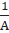----- equation 2Combining equation 1 and 2 we get-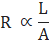So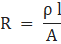Where ρ is proportionality constant and is called specific resistance or resistivity.Question 7.How do you verify that resistance of a conductor is proportional to the length of the conductor for constant cross-section area and temperature?Answer:A circuit is prepared by using a cell, key, ammeter as shown in below figure.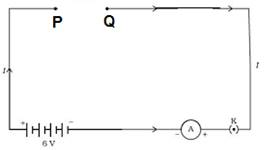Iron wires of different lengths but same cross-sectional area were taken. Connect one wire between terminals P and Q. The ammeter reading was noted. The same procedure was repeated for other wires. We will observe that current decreases with increase in the length of wire. Resistance increases with increase in length and current decreases. Thus we can prove resistance of a conductor is proportional to the length of the conductor for constant cross-section area and temperature.Question 8.Explain Kirchhoff's laws with examples.Answer:Kirchhoff’s junction law states that at any junction point in a circuit where the current can divide the sum of currents into the junction is equal to the sum of currents leaving the junction.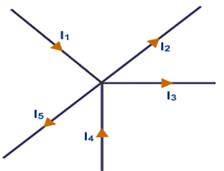Here I1, I4 are currents into the junction whereas I2, I5, I3 are currents out of a junction. So I1 + I4 = I2 + I3 + I5Kirchhoff’s loop law- The algebraic sum of increases and decreases in potential difference across various components of a closed circuit loop must be zero.For loop ACDBA-V2 + I2R2 – I1R1 + V1 = 0For loop EFDCE-(11 + 12) R3 –I2R2 + V2 = 0Question 9.What is a value of 1 KWH in Joules?Answer:1 KWH = 1 KW x 1 hour= 1000 W x 60 x 60 sec= 36 x 105 W.secSo 1KWH = 3.6 x 106 joulesQuestion 10.Explain overloading of the household circuit.Answer:In our house, the live wires entering our house meter have a potential difference of 240 V and minimum-maximum limit of current drawn from mains is 5-20 A. if the current drawn exceeds 20 A it causes overheating which may cause a fire. It happens mostly when many appliances are connected to a circuit. This is called overloading.Question 11.Why do we use fuses in household circuits?Answer:Electric fuses are connected in household circuits to prevent damages due to overloading. It is a thin wire with a low melting point when current flow exceeds the limit the fuse wire gets heated up and melts. Thus circuit breaks and current flow stops. Thus appliances are saved from being damaged.Question 12.Deduce the expression for the equivalent resistance of three resistors connected in series.Answer: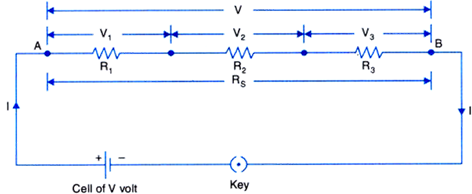Series combination of resistors: If a number of resistors are joined end to end so that the same current flows through each of them in succession, then the resistors are said to be connected in series.As shown in the figure, consider three resistors R1, R2, R3 connected in series. Suppose a current I flows through the circuit when a cell of V volt is connected across the combination.By Ohm’s law, the potential differences across the three resistors will be,V1 = IR1, V2 = IR2, V3 = IR3If Rs be the equivalent resistance of the series combination, then on applying a potential difference V across it, the same current I must flow through it.Therefore,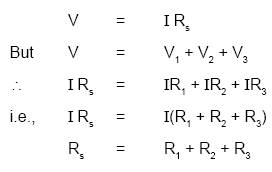Question 13.Deduce the expression for the equivalent resistance of three resistors connected in parallel.Answer: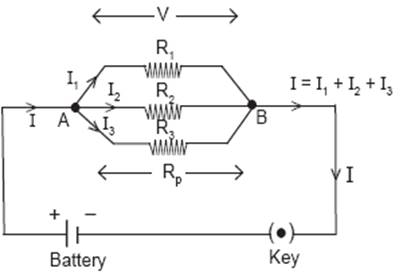The parallelcombination of resistors: If a number of resistors are connected in between two common points so that each of them provides a separate path for current, then they are said to be connected in parallel.As shown in the figure, consider three resistors R1, R2, R3 connected in parallel.Suppose a current I flows through the circuit when a cell of voltage V is connected across the combination. The current I at point A is divided into three parts I1, I2, I3 through the resistors R1, R2, R3 respectively. These three parts recombine at point B to give the same current I.∴ I = I1 + I2 + I3As all the three resistors have been connected between the same two points A and B, voltage V across each of them is same. By Ohm’s law,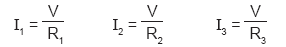If RP be the equivalent resistance of parallel combination, then,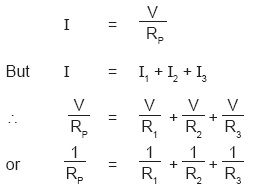Question 14.Silver is a better conductor of electricity that copper. Why do we use copper wire for conduction of electricity?Answer:Though silver is the best conductor of electricity it is very expensive so we use copper wire for conduction of electricity. Copper is also more readily available and cheaper, affordable as compared to silver so we use copper wire instead of silver wires for conduction of electricity.Question 15.Two bulbs have ratings 100 W, 220V and 60 W. 220 V. Which one has the greater resistance?Answer:We know P = V2/RSo R = V2/PFirst bulbR =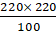= 484 ohmSecond bulbR =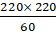= 806.66 ohmSo second bulb with 60 W, 220 V has greater resistance.Question 16.Why don't we use series arrangement of electrical appliances like bulb, Television, fan and others in domestic circuits?Answer:The current is same throughout the circuit in series combination. So we cannot connect various electrical appliances as they draw different amount of current to operate. Secondly if one appliance connect in a series circuit fails other appliances will also not work as circuit will break. So we prefer parallel arrangement of electrical appliances like bulb, Television, fan and others in domestic circuits.Question 17.A wire of length 1m and radius 0.1 mm has a resistance of 100Ω. Find the resistivity of the material.Answer:Given - R = 100 ohm;l = 1 mr = 0.1 mm = 0.0001 mTo find resistivity = ?We know R = ρ l/Aρ =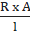where A = cross sectional areaA = πr2A =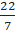x 0.0001 x 0.0001A = 0.00000022/7 m2ρ =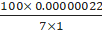Resistivity = 3.142 e-6 ohm.mQuestion 18.Why do we consider tungsten as a suitable material for making the filament of a bulb?Answer:Tungsten has high resistance and high melting point. So when the filament will glow it can withstand high temperature and emits light without getting melted. So tungsten is considered as a suitable material for making the filament of a bulb.Question 19.Are the head lights of a car connected in series or parallel? Why?Answer:The head lights of the car are connected in parallel so that if one does not work other will continue to work. If one of the lights get fused then other will work without causing any difficulty.Question 20.Why should we connect electrical appliances in parallel in a household circuit? What happens if they are connected in series?Answer:We should connect electrical appliances in parallel so that each appliance would get full voltage. If one appliance is faulty, other appliance will continue working. Each appliance will get the desired current according to their resistance.If they will be connected in a series resistance will be more so the current flow will decrease. The same amount of current will flow through every appliance which is not required. All appliances should have to use simultaneously even though we do not need them. We cannot use independent switches for individual appliances.Question 21.Suppose that you have three resistors each of value 30 Ω. How many resistors can you obtain by various combinations of these three resistors? Draw diagrams in support of your predictions.Answer:We can connect all three in series.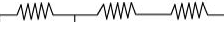We can connect all three in parallel.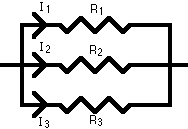We can connect two in series and one parallel to them.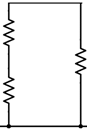We can connect two in parallel and one in series with them.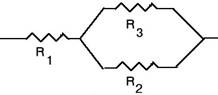Question 22.State Ohm's law. Suggest an experiment to verify it and explain the procedure.Answer:Ohm’s law:The current through a conductor element is proportional to the potential difference applied between its ends provided the temperature remains constant.V = IRAim: To show that the ratio of V/I is a constant for a conductor.Materials required: 5 dry cells of 1.5 V each, conducting wires, an ammeter, a voltmeter, thin iron spoke of length 10 cm, LED and key.Procedure: i) Connect a circuit as shown in the figure.ii) Solder the conducting wires to the ends of the iron spoke and close the key.iii) Note the reading of ammeter (current) and voltmeter (potential difference) and tabulate them.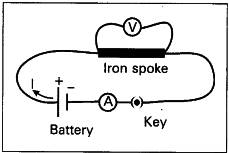iv) Now connect two cells in the circuit and note the respective readings of ammeter and voltmeter in the above table.v) Repeat the above procedure using three cells, four cells, and five cells respectively.vi) Record the values of potential difference and current corresponding to each case in the table.vii) Find V/I for each set of values.viii) We notice that V/I is constant.ix) From this, we can conclude that potential difference between the ends of the iron spoke is directly proportional to the current passing through it. This is Ohm's law.Question 23.Take a battery and measure the potential difference. Make a circuit and measure the potential difference when the battery is connected in the circuit. Is there any difference in potential difference of battery?Answer:When battery is not connected in a circuit its chemicals are not used up. So it gives a different reading of potential difference. But when the battery is used in a circuit the voltage slowly decreases as it is consumed, chemical energy stored in it converts to electrical energy. So we obtain a difference in potential difference before and after using a battery in the electrical circuit.Question 24.Measure the resistance of a bulb (filament) in open circuit with a multi-meter. Make a circuit with elements such as a bulb, a battery of 12V and key in series. Close the key. Then again measure the resistance of the same bulb (filament) every 30 seconds. Record the observations in a proper table. What can you conclude from the above results?Answer:We can measure current in ammeter and voltage using voltmeter every 30 seconds. We will found the resistance by applying formula R = V/I i.e. Ohm’s law for every readings of ammeter and voltmeter. We will see resistance is constant. Since resistance is constant so it represents Ohm’s law. If we plot a graph taking voltage in Y axis and current in X axis, a straight line will be obtained indicating that resistance is constant and it obeys Ohm’s law.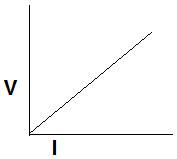Question 25.Draw a circuit diagram for a circuit in which two resistors A and B are connected in series with a battery and a voltmeter is connected to measure the potential difference across the resistor A.Answer: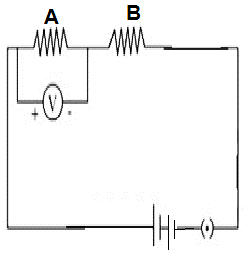In the above circuit diagram there are two resistors A and B which are connected in series. To resistor A voltmeter is connected in parallel. A battery and a key is also connected. When key is switched on current will flow across resistors. If key is off no current flows across both the resistors.Question 26.How can you appreciate the role of a small fuse in house wring circuit in preventing damage to various electrical appliances connected in the circuit?Answer:Electric fuses are connected in household circuits to prevent damages due to overloading. It is a thin wire with low melting point, when current flow exceeds the limit the fuse wire gets heated up and melts. Thus circuit breaks and current flow stops. Thus appliances and household wiring are saved from being damaged.Question 27.In the figure Q-26 the potential at A is ________ when the potential at B is zero.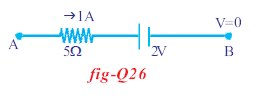Answer:At point A potential difference V = IRSo V = 1 x 5 = 5 VQuestion 28.Observe the circuit and answer the questions given below.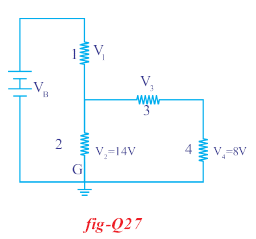i Are resistors 3 and 4 in series?ii. Are resistors 1 and 2 in seriesiii. Is the battery in series with any resistor?iv. What is the potential drop across the resistor 3?v. What is the total emf in the circuit if the potential drop across resistor 1 is 6V?Answer:i. Yes, resistors 3 and 4 are in series as they are connected end to end.ii. Yes, resistors 1 and 2 are in series.iii. Yes, the battery is in series with resistors 1 and 2.iv. Resistors 3 and 4 are in series and combination of both are in parallel with 2 so V3 + V4 = V2V3 + 8 = 14V3 = 6 VSo potential drop across resistor 3 is 6 Vv. Total emf = 14 + 6 = 20 VQuestion 29.If the resistance of your body is 100000 Ω that would be the current that flows in your body when you touch the terminals of a 12V battery?Answer:Given R = 100000 ohmV = 12 VTo find IWe know V = IRSo I = V/RI = 12/100000I = 0.00012 AQuestion 30.A uniform wire of resistance 100 Ω melted and recast into wire of length doubles that of the original. What would be the resistance of the new wire formed?Answer:Given R of original wire = 100 ohmWhen length is l, R = 100 ohmWhen length is 2l, R’ = ?We know R = ρ l/AR’ = ρ 2l/AR’ = 2 ρ l/AR’ = 2R [ since ρ l/A = R]So R’ = 2 x 100R’ = 200 ohmQuestion 31.A house has 3 tube lights, two fans and a Television. Each tube light draws 40W. The fan draws 80W and the Television draws 60W. On the average, all the tube lights are kept on for five hours, two fans for 12 hours and the television for five hours every day. Find the cost of electric energy used in 30 days at the rate of Rs. 3.00 per Kwh.Answer:1 tube light consume power = 40 WSo 3 tube lights will consume = 3 x 40 = 120 WThey are kept on for 5 hours so energy consumed = 120 x 5 = 600 Wh1 fan consume power = 80 W2 fans will consume = 80 x 2 = 160 WThey work for 12 hours, so energy consumed = 160 × 12 = 1920 WhTelevision consumes power = 60 WIt works for 5 hours, so energy consumed = 60 x 5 = 300 WhTotal energy consumed in 1 day = 600 + 1920 + 300 = 2820 Wh = 2.820 KwhConsumption for energy in 30 days = 30 x 2.820 KWhCost is Rs 3 for 1 kWhSo total amount = 3 x 30 x 2.820 = Rs 253.80

PDF FILE TO YOUR EMAIL IMMEDIATELY PURCHASE NOTES & PAPER SOLUTION. @ Rs. 50/- each (GST extra)

HINDI ENTIRE PAPER SOLUTION

MARATHI PAPER SOLUTION

SSC MATHS I PAPER SOLUTION

SSC MATHS II PAPER SOLUTION

SSC SCIENCE I PAPER SOLUTION

SSC SCIENCE II PAPER SOLUTION

SSC ENGLISH PAPER SOLUTION

SSC & HSC ENGLISH WRITING SKILL

HSC ACCOUNTS NOTES

HSC OCM NOTES

HSC ECONOMICS NOTES

HSC SECRETARIAL PRACTICE NOTES

# 2019 Board Paper Solution

HSC ENGLISH SET A 2019 21st February, 2019

HSC ENGLISH SET B 2019 21st February, 2019

HSC ENGLISH SET C 2019 21st February, 2019

HSC ENGLISH SET D 2019 21st February, 2019

SECRETARIAL PRACTICE (S.P) 2019 25th February, 2019

HSC XII PHYSICS 2019 25th February, 2019

CHEMISTRY XII HSC SOLUTION 27th, February, 2019

OCM PAPER SOLUTION 2019 27th, February, 2019

HSC MATHS PAPER SOLUTION COMMERCE, 2nd March, 2019

HSC MATHS PAPER SOLUTION SCIENCE 2nd, March, 2019

SSC ENGLISH STD 10 5TH MARCH, 2019.

HSC XII ACCOUNTS 2019 6th March, 2019

HSC XII BIOLOGY 2019 6TH March, 2019

HSC XII ECONOMICS 9Th March 2019

SSC Maths I March 2019 Solution 10th Standard11th, March, 2019

SSC MATHS II MARCH 2019 SOLUTION 10TH STD.13th March, 2019

SSC SCIENCE I MARCH 2019 SOLUTION 10TH STD. 15th March, 2019.

SSC SCIENCE II MARCH 2019 SOLUTION 10TH STD. 18th March, 2019.

SSC SOCIAL SCIENCE I MARCH 2019 SOLUTION20th March, 2019

SSC SOCIAL SCIENCE II MARCH 2019 SOLUTION, 22nd March, 2019

XII CBSE - BOARD - MARCH - 2019 ENGLISH - QP + SOLUTIONS, 2nd March, 2019

# HSCMaharashtraBoardPapers2020

(Std 12th English Medium)

HSC ECONOMICS MARCH 2020

HSC OCM MARCH 2020

HSC ACCOUNTS MARCH 2020

HSC S.P. MARCH 2020

HSC ENGLISH MARCH 2020

HSC HINDI MARCH 2020

HSC MARATHI MARCH 2020

HSC MATHS MARCH 2020

# SSCMaharashtraBoardPapers2020

(Std 10th English Medium)

English MARCH 2020

HindI MARCH 2020

Hindi (Composite) MARCH 2020

Marathi MARCH 2020

Mathematics (Paper 1) MARCH 2020

Mathematics (Paper 2) MARCH 2020

Sanskrit MARCH 2020

Sanskrit (Composite) MARCH 2020

Science (Paper 1) MARCH 2020

Science (Paper 2)

Geography Model Set 1 2020-2021

MUST REMEMBER THINGS on the day of Exam

Are you prepared? for English Grammar in Board Exam.

Paper Presentation In Board Exam

How to Score Good Marks in SSC Board Exams

Tips To Score More Than 90% Marks In 12th Board Exam

How to write English exams?

How to prepare for board exam when less time is left

How to memorise what you learn for board exam

No. 1 Simple Hack, you can try out, in preparing for Board Exam

How to Study for CBSE Class 10 Board Exams Subject Wise Tips?

JEE Main 2020 Registration Process – Exam Pattern & Important Dates

NEET UG 2020 Registration Process Exam Pattern & Important Dates

How can One Prepare for two Competitive Exams at the same time?

8 Proven Tips to Handle Anxiety before Exams!